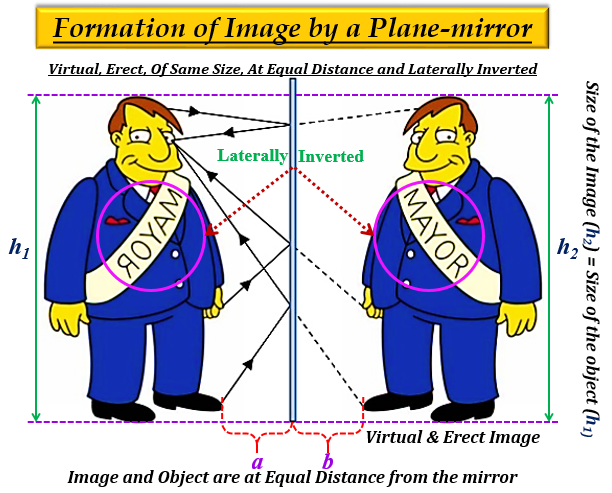"">

# Explain the characteristics of Image formed by a Plane Mirror"."

Characteristics of the image formed by a plane mirror :
1. The image formed by a plane mirror is virtual (behind the mirror)

2. The image formed by a plane mirror is erect (upright).

3. The size of the image formed by a plane mirror is the same as that of the size of the object.
For example- If an object is 15 cm high, then the image of this object will also be 15 cm high.

4. The distance of the image formed by the plane mirror, is equal to the distance of the object from the mirror.
For example- Suppose, an object is placed at 10 cm in front of a plane mirror then its image will be at 10 cm behind the plane mirror.

5. The image formed by a plane mirror is laterally inverted, means the right side of the object appears as the left side of its image and vice-versa (left side of the object appears as the right side of its image).

Therefore, in short, the image formed by a plane mirror is virtual, erect (upright), of the same size, at the same distance and laterally inverted.Updated on: 10-Oct-2022

235 Views